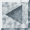Verbose C4.5rules Manual PageVERBRULES(1)

NAME

A guide to the verbose output of the C4.5 production rule generator

DESCRIPTION

This document explains the output of the program C4.5rules when it is run with the verbosity level (option v) set to values from 1 to 3. C4.5rules converts unpruned decision trees into sets of pruned production rules. Each set of rules is then sifted to find a subset of the rules which perform as well or better on the training data (see c4.5rules(1)).

RULE PRUNING

Verbosity Level 1

A decision tree is converted to a set of production rules by forming a rule correŁ sponding to each path from the root of the tree to each of its leaves. After each rule is extracted from the tree, it is examined to see whether the rule can be generalised by dropping conditions.

For each rule, the verbose output shows the following figures for the rule as it stands, and for each of the rules that would be formed by dropping any one of the conditions:

 Miss - number of items misclassified by the rule Hit - number of items correctly classified by the rule Pess - the pessimistic error rate of the rule (i.e. 100*(misses+1)/(misses+hits+2)) Gain - the information gain of the rule Absent condition - the condition being ignored

If there are any conditions whose deletion brings about rules with pessimistic error rate less than the default error rate, and gain greater than that of the rule as it stands, then the one of these with the lowest pessimistic error rate is dropped. When this happens, the message:

eliminate test d

is given and the new rule without condition d is examined, and so on.

When the rule has been pruned, either the rule is displayed, or the message:

duplicates rule n

is given, where n is an identical rule already produced, and so the new rule is not added, or the message:

too inaccurate

is given, indicating that the pessimistic error rate of the pruned rule is more than 50%, or more than the proportion of the items that are of the rule's class, and so the rule is not added.

RULE SIFTING

Verbosity Level 1

The set of pruned rules for each class is then examined. Starting with no rules in the ruleset, the following process is repeated until no rules can be added or dropped.

1. If there are rules whose omission would not lead to an increase in the number of items misclassified, then the least useful of these is dropped.
2. Otherwise, if there are rules which lead to a decrease in the number of items misclassified, then the one with the least counterexamples is added.

This is shown in the output as:

 Action - the number of the rule added or dropped Change - the advantage attributable to the rule Worth - the included rules for this class as:

n1[n2|n3= r1]

with:

 n1 - the rule number n2 - the number of items that correctly fire this rule and are not covered by any other included rule n3 - the number of items that incorrectly fire this rule and are not covered by any other included rule r1 - the advantage attributable to the rule

After the rules have been sifted, the number of items of each class that are not covered by any rules is shown, and the default class is set to the class with the most uncovered items.

Verbosity Level 2

When sifting rules for a particular class, the Worth of each rule which is for that class but not included in the ruleset, is shown at each stage of the process.

RULE SORTING

Verbosity Level 1

The rules that are left are then sorted, starting with those that are for the class with the least number of false positives. The verbose output shows the number of false positives for each class (i.e., the number of items misclassified as being of this class). Within a class, rules with the greatest advantage are put first.

RULESET EVALUATION

Verbosity Level 3

When evaluating a ruleset, shown are the attribute values, given class and class given by the ruleset for each item that is misclassified.